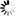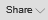Valuation tables

mohammed ibrahim View Profile My Other Post
Architect-Engineer-Valuer

Construction of Valuation Tables: Valuation tables are means of making conversion of money spent or received at different times into a common time scale. Interest is the money paid for the use of money. The money which is lent is the principal. The money is used in compound interest factor. These assumptions provide the basis for valuation tables. In assessing the leasehold interests or leased fee interests valuers use present value, year’s purchase and reversionary, etc. valuation tables. To work manually is time consuming and valuation tables are ready reckoner and easy to refer.

Single Rate Compounding and Discounting Tables:

Present value of INR 1/- at 4% (Rate) compound interest in five (05) years will fetch INR. 1.2166/-.

I.            Year 1=(INR1+0.04) or (INR 1.04)1; Year 2=(INR 1.04) x (INR 1.04)=INR 1.0816 or INR (INR 1.04)2; Year 3=(INR 1.04) x (INR 1.04) x (INR 1.04) = INR 1.1248 or (1.04)3 = INR 1.12486; Year 4= (INR 1.04) x (INR 1.04) x (INR 1.04) x (INR 1.04) = INR 1.1698 or INR (1.04)4 = 1.1698; Year 5=(INR 1.04) x (INR 1.04) x (INR 1.04) x (INR 1.04) x (INR 1.04) = 1.2168 or (INR 1.04)5 = 1.2166. This is Single payment future value of INR 1/- Say “SPCAF”. (Single Payment Compound Amount Factor).

II.          Single payment present value or Reversionary value is: 1/SPCAF = 1/1.2168 = 0.8219.This Single Payment Present Value of INR 1/-, Say “SPPWF” (Single Payment Present worth Factor).

III.        Annual Sinking Fund Factor: Rate/ (SPCAF-1) = 0.04/ (1.2166 – 1) = 0.04/0.2166 = 0.1846. Say “USSFDF”. (Uniform Series of Sinking Fund Deposit Factor).

IV.        Present Value of INR 1/- per annum: SPCAF-1/ (SPCAF X Rate) = 1.2166 – 1 /1.216 x 0.04 = 0.2166/1.2166 x 0.04 = 4.451 Say “USPWF” (Uniform Series of Present worth Factor) or Year’s Purchase (Y. P.).

Dual Rate Valuation Tables: Assuming the building is constructed by the lessee, will revert to the lessor after the expiry of the lease period, the lessee has to redeem his investment by investing in an Annual Sinking Fund (A. S. F). Annual Sinking Fund Rate will be different from prime interest rate. Assuming 4 percent on capital and 3 percent (A. S. F. factor) on redemption of capital for a period of 5 years:

SPCAF for 5 years at 3 percent = (1.03)5 = 1.159274; A.S.F: Rate SF/Sum (SPCAF-1) = 0.03/1.159-1) = 0.03/0.159 = 0.18835; USPWF: 1/sum (Rate + A.S.F) = 1/0.04+0.18835 = 4.3791. Year’s purchase: 4.3791.

Instead of working manually, online Valuation Tables (Single and Dual Rates) compiled by the author in MS Excel can be referred, which is attached below:

Please Find Valuation Tables (Compound Interest Factors)

 Tables - I Single Rate Tables - II Dual Rate Tables - III Present Value of a Reversion to a perpetuity (Wood’s Tables) (Same Dual Rates) Tables - IV Present Value for deferred Period (Different   Dual Rates)

A. M. Ibrahim

Attached File : 966690578 am ibrahim valuation tables 1.xls downloaded 127 times

Your are not logged in . Please login to post repliesSearch Forum:

×